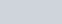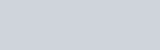# Best MATLAB Online Courses, Training with Certification-2022 Updated

Now a days MATLAB is having more demand in market. Here we listed Best MATLAB Online Courses and this is the right place to select best course. Concept of MATLAB, about simulink programming, how to develop programs by using MATLAB, fundamentals of MATLAB, how to interface with compute operating system, how to build mobile applications, recommender systems, techniques used in MATLAB, email filtering, computer programming applications, categorization, layouts and etc. all this concepts are covered in this courses. In the world we are seeing many MATLAB online courses. From all the courses, out expert panel handpicked some of the best MATLAB online courses and those are listed below.

## #1 Introduction to Programming with MATLAB by Vanderbilt University-CourseraAkos Ledeczi and Mike Fitzpatrick are the professors in Vanderbilt University. In this course both are going to teach you about how to develop programs by using MATLAB. This course is created by Vanderbilt University. To learn this course no need of any previous knowledge. This course will more useful for engineers. MATLAB is one of the programming language, by using this language you can write moderate-size programs. You can also use different programming languages in MATLAB. By learn this course you will become expert in MATLAB. It will take nine weeks to complete this course.
Key points:

• In first week and second week you will learn about course pages and also about MATLAB environment and user interfaces. Here you will learn about semantics and syntaxes. And know how to plot the graphs in MATLAB.
• In third week and fourth week they will teach you about matrices and operations of MATLAB and also about functions of MATLAB. Here you will learn how to extract, define and combine the matrices.
• In fifth week you will learn about how the toolbox is used in MATLAB programs. Here you will learn about the polymorphism.
• In sixth and seventh week they will teach about if statements and also about loop in MATLAB. You will learn how to use the logical operators and relational operators.
• In eighth week you will learn about how many data types are used in MATLAB.
• In ninth week they will teach you about how to input the files and also about how to output the files.

Rating : 4.8 out of 5
You can Signup here <=>

## #2 Become a Good Matlab Programmer in 30 days-Udemy

Key points:

• By learning this course you will learn how to write your own MATLAB programs.
• He will teach you about how to build technical project and also about engineering projects.
• At end of the course you will learn about how to use all programming skills in MATLAB.
• Hossein will also teach you how to plot the diagrams and graphs in MATLAB.
• You will learn about in MATLAB, how to use Matrices and you will also learn about conditions and loop which are use in MATLAB.

Rating : 4.3 out of 5
You can Signup here <=>

## #3 Master MATLAB through Guided Problem Solving-Udemy

Key points:

• They will teach you by using syntax rules how to solve the problems in MATLAB.
• You will also learn about how to build real world applications in MATLAB.
• They will also teach you about matrix decompositions, non-linear and linear model-fitting and etc.
• You will also learn about data animations to draw 2D and 3D diagrams in MATLAB.

Rating : 4.6 out of 5
You can Signup here <=>

## #4 Learn MATLAB and Simulink Programming-Udemy

Key points:

• You will also learn about MATLAB commands and also about how to use them.
• By learning this course you will learn how to build your own Simulink models and also about how to simulate them.
• Jordan will also teach you about conditional statements and also about programming loops.
• You will also learn about Simulink features and also about Matrix Commands. Here you will learn how to create the scripts and how to run that scripts in the MATLAB.
• Instructors will also teach you about vector arithmetic, matrices arithmetic and you will know how to access the individual elements etc.

Rating : 4.2 out of 5
You can Signup here <=>

## #5 Complete MATLAB Tutorial: Go from Beginner to Pro-Udemy

Nouman Azam is an MATLAB professor and also an instructor on Udemy. He will teach about how MATLAB is used in routine daily life activities. He will also teach about MATLAB programming, data analysis and etc. he will explain about by using MATLAB what are the techniques used in Machine learning like, text categorization, recommender systems, email filtering and etc. in this course he is going to teach about complete concept of MATLAB. You will also learn about problems and projects by doing more practice. To learn this course 3K+ students enrolled their names. To learn this course no need of any previous knowledge and no need of any previous experience. MATLAB is one of the most fundamental programming language. In this course you will also learn about some other programming languages like, Java, C++ and C. he will also explain you complete operations of MATLAB and also about how to import the data in to MATLAB. It is having 16 downloadable resources, 14 articles, 9.5hours on demand videos and it is also having full lifetime access.
Key points:

• By learn this course you will know how to solve the problems by using MATLAB in different ways.
• He will teach you how to solve the equations and also about by using different methods how to do manipulate matrices and math operations.
• By learning this course you will learn how to write you own code and also about how to do data analysis.
• You will learn about by using different programming skills, how to translate own logic and complex problems in to MATLAB code.

Rating : 4.2 out of 5
You can Signup here <=>

Conclusion: